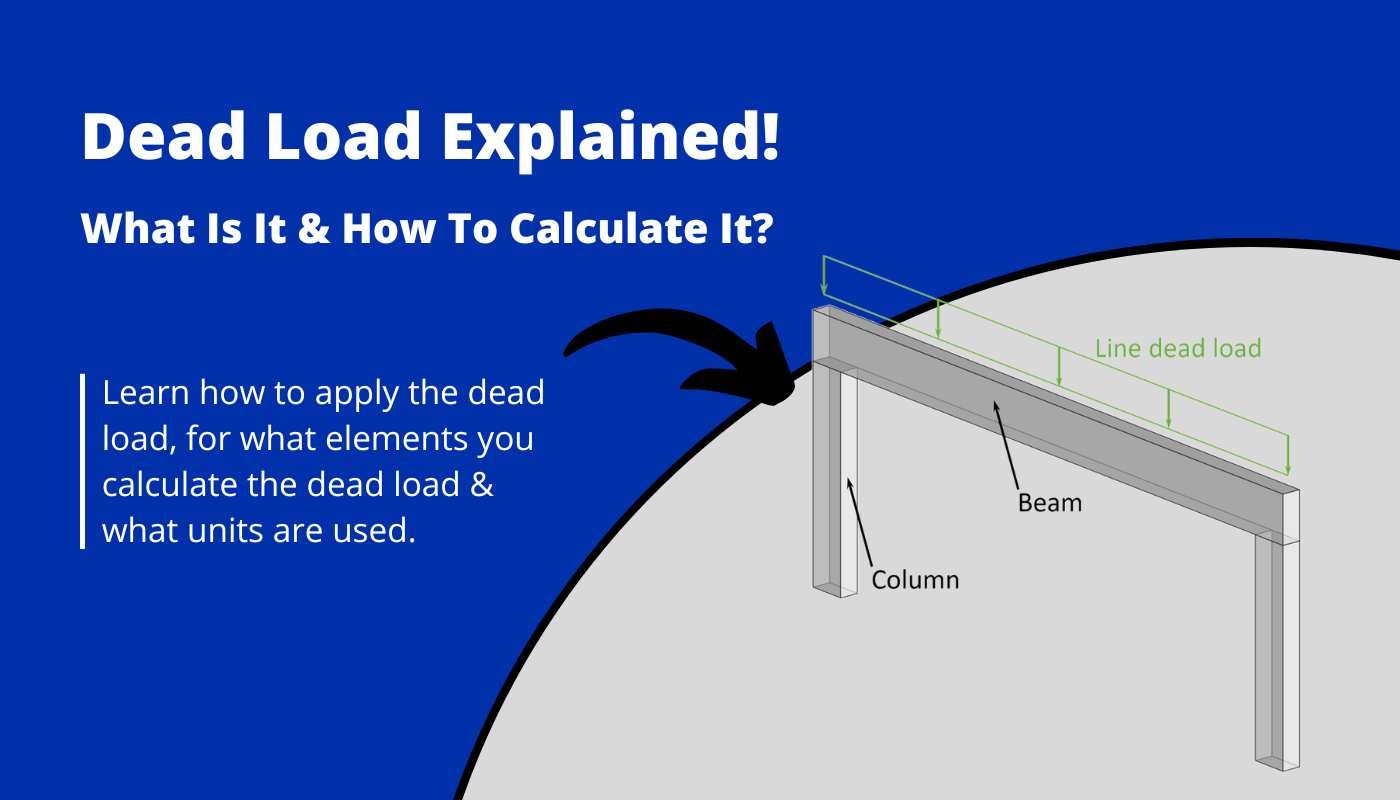# Dead Load – What is It and How to Calculate It?Last updated: June 6th, 2023

Structural loads are the basics of structural design. Without defining what loads act on a building or structure, the engineer can’t verify the structural element.

The dead load is one of many loads that need to be considered in a structural design.

Other loads that act on buildings are:

Let’s get started.

The dead load represents permanent loads, such as the self-weight of structural and non-structural building materials. The self-weight of a concrete slab, a timber truss roof and windows are examples of the dead load. The weight is calculated and then applied to the structural member that carries it.

How the dead load for different elements is calculated and then applied to different static systems, will be explained in later sections🔥.

So stick with us.

How the dead load is calculated depends on the structural element that needs to withstand the load.

For example, the dead load of a slab is usually calculated as an Area load ($kN/m^2$) because the slab itself – 2D static element – needs to carry the load.

On the other hand, the dead load applied on 1D static elements like beams, columns, rods, etc. are usually either line ($kN/m$) or point loads ($kN$).

So let’s have a look at how to calculate the different dead load types.

$$g_k = \mbox{Density of element} \cdot \mbox{thickness}$$

The Area dead load of a concrete slab with a density of 2400 $\frac{kg}{m^3}$ and a thickness of 18 cm is calculated as

$$g_k = 2400 \frac{kg}{m^3} \cdot 0.18m = 432 \frac{kg}{m^2}$$

Now, as engineers don’t use kg in their calculations any more, the unit needs to be transferred from kg to kN [Kilonewton].

To get kN you need to multiply the result by 0.0098. Or if you are a bit lazier, in most structural engineering cases it’s enough to multiply it by 0.01

$$g_k = 432 \frac{kg}{m^2} = 4.24 \frac{kN}{m^2}$$

An example where the dead load is calculated as a line load ($kN/m$) are beams. So in this case, we are calculating the dead load of a wood beam.

$$g_k = \mbox{Density of element} \cdot \mbox{Cross-section width} \cdot \mbox{Cross-section height}$$

The line dead load of a wooden GL24h beam with a density of 420 $\frac{kg}{m^3}$, Cross-section width of 10cm and a Cross-section height of 20cm is calculated as

$$g_k = 420 \frac{kg}{m^3} \cdot 0.2m \cdot 0.1m = 8.4 \frac{kg}{m}$$

As for the Area load, the line load also has to be transferred from kg to kN.

$$g_k = 8.4 \frac{kg}{m} = 0.08 \frac{kN}{m}$$

An example where the dead load is calculated as a point load ($kN$) are columns. So in this case, we are calculating the dead load of a wood column.

$$g_k = \mbox{Density of element} \cdot \mbox{Cross-section width} \cdot \mbox{Cross-section height} \cdot \mbox{Column length}$$

The point dead load of a wooden GL24h column with a density of 420 $\frac{kg}{m^3}$, Cross-section width of 50cm, a Cross-section height of 50cm and a length of 3m is calculated as

$$g_k = 420 \frac{kg}{m^3} \cdot 0.5m \cdot 0.5m \cdot 3m = 315 kg$$

As for the line load, the point load also has to be transferred from kg to kN.

$$g_k = 315 kg = 3.1 kN$$

Here are some examples of building materials which need to be considered in the dead load if used:

• MEP equipment
• Staircases
• Steel, concrete & wood beams
• Elevators
• Glass windows and panels
• Timber & concrete slabs
• Insulation
• Roofing
• Bricks
• Tiles
• Ventilation ducts
• Steel, concrete & wood columns
• Heating systems
• Solar panels
• Garage ramps
• etc.

Just to mention, a few 😎.

As already explained above, depending on which structural element the building element is attached to an area, line or point load needs to be calculated from the density.

Some product manuals include the information of how much the element weighs.

Now that we know how to calculate the dead load and its different types, we have to take a look at how to apply it to structural members.

In most cases the dead load is applied to a horizontal element like for example a slab, balcony, flat roof, stairs.

And in that case the dead load can simply be applied vertically, as in the next picture, where a floor slab is supported on 3 edges by walls.

Now, it gets a bit more tricky when we need to apply the dead load to an inclined structural member like a rafter roof. We wrote an extensive article about loads on roof structures, and you can check it out here.

The load direction is z-axis downwards ⬇️, while the distribution follows the inclination of the roof.

## 👩‍🏫 Unit

The unit of the dead load in Europe is one of the following:

• Area load: $kN/m^2$ = Kilonewton per square meter
• Line load: $kN/m$ = Kilonewton per meter
• Point load: $kN$ = Kilonewton

## 🤝 Conclusion

Now, that you got an understanding of what the dead load is and how it is used, it’s time to look at other loads like

Because there are always multiple loads acting on a structural element. Considering these different loads in the structural design is done by setting up Load Combinations with safety factors.

Once all load cases and combinations are set up, the structural elements can be designed. We have already written a lot of guides on how to design structural elements. Check them out.

## Similar Posts

1.google lens app says:

Great article.

1.Laurin Dominik says:

Hi!
1.Laurin Dominik says: